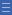## Featured resource

Home > Topdrawer > Mental computation > Activities > Partial arrays

# Partial arrays

Partial arrays

Array structures are ways to represent factors. In this activity, students are given the number of tiles in each row and column and are asked to find the total number of tiles needed to construct the rectangle.

Factors are pairs of counting numbers that multiply to give another counting number. They can be represented by arrays.

Make a partial array on the board (e.g. 6 by 5). Ask the students to predict the number of tiles they think were used to make the complete array and how they might work it out.

Students play a game of 'How many tiles do I need to pave the floor?'

Variation: Students tell a partner the number of tiles in each row and the number of rows in the array. Restrict the numbers to single digits.

Strategies such as skip counting or double count suggests students are attending to the row and column structure. This also suggests they recognise and are treating the number of tiles in each row as composite units rather than as ones.

More advanced thinking is evident when a student derives the total number of tiles (product) from a known multiplication fact.

For example, a student might use knowledge of 5 $$\times$$ 5 = 25 to work out the number of tiles for the 6 rows of 5 array. Invite students who use this type of thinking to share with the class. Challenge others to think of known facts as a starting point for finding the total number of tiles.

Yes

Yes

Name Class SectionYear 2: Recognise and represent multiplication as repeated addition, groups and arrays Infobox 3Year 3: Recall multiplication facts of two, three, five and ten and related division facts Infobox 3Year 4: Recall multiplication facts up to 10 x 10 and related division facts Infobox 3Source Infobox 3